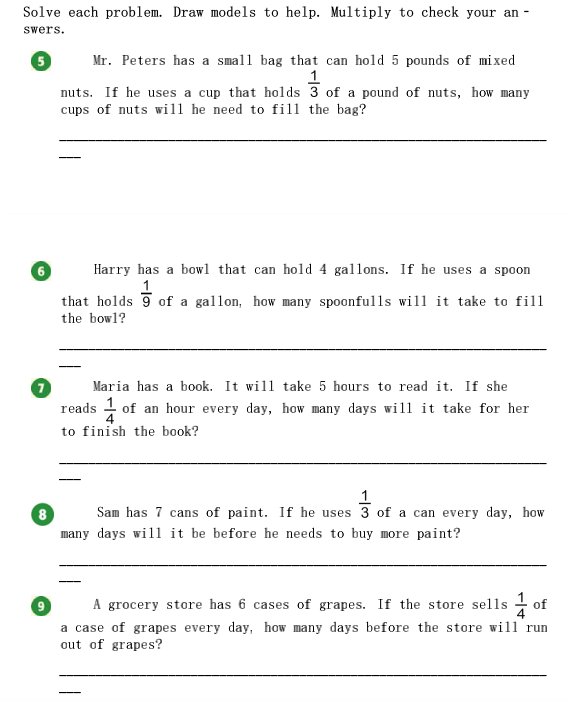### ¿Todavía tienes preguntas de matemáticas?

Pregunte a nuestros tutores expertos
Algebra
PreguntaSolve each problem. Draw models to help. Multiply to check your answers.

(5). Mr. Peters has a sma11 bag that can hold $$5$$ pounds of mixed nuts. If he uses a cup that holds $$\frac { 1 } { 3 }$$ of a pound of nuts, how many cups of nuts will he need to fill the bag?

(6) Harry has a bowl that can hold $$4$$ gallons. If he uses a spoon that holds $$\frac { 1 } { 9 }$$ of a gallon, how many spoonfulls will it take to fill the bowl?

(7) Maria has a book. It will take $$5$$ hours to read it. If she reads $$\frac { 1 } { 4 }$$ of an hour every day, how many days will it take for her to finish the book?

(8) ham $$7$$ cans of paint. If he uses $$\frac { 1 } { 3 }$$ of a can every day, how many days will it be before he needs to buy more paint?

(9) A grocery store has $$6$$ cases of grapes. If the store sel1s $$\frac { 1 } { 4 }$$ of a case of grapes every day, how many days before the store wil1 run out of grapes?

5) $$\frac{5}{\frac{1}{3} }$$ = 5 x 3 = 15 , 6) $$\frac{4}{\frac{1}{9} }$$ = 4 x 9 = 36 , 7) $$\frac{5}{\frac{1}{4} }$$ = 5 x 4 = 20 , 8) $$\frac{7}{\frac{1}{3} }$$ = 7 x 3 = 21 , 9) $$\frac{6}{\frac{1}{4} }$$ = 6 x 4 = 24